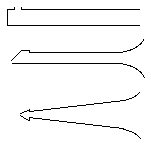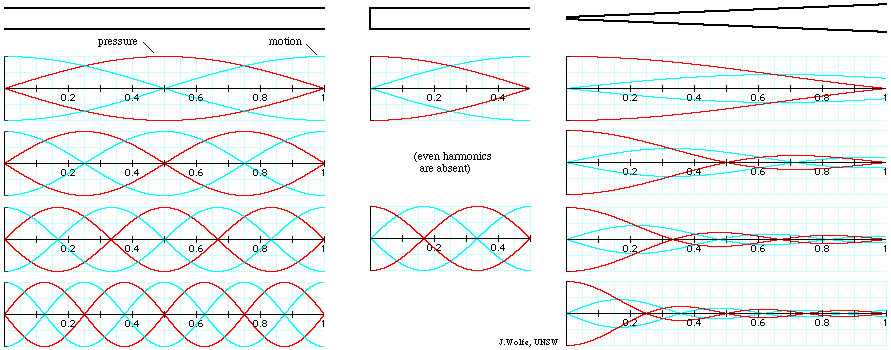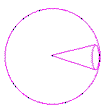# Pipes and Harmonics

Why do closed conical bores have the same set of resonances as open cylindrical bores of the same length, whereas closed cylindrical bores of the same length have only odd harmonics starting one octave lower?

The bores of three woodwind instruments are sketched below. The diameters are exaggerated. The flute (top) and clarinet (middle) are nearly cylinders. The oboe (right) is nearly conical (as are the saxophone and bassoon). The clarinet is about the same length as the flute, but plays nearly an octave lower. The oboe is closed like the clarinet, but its range is close to that of the flute.Here we give an answer to this frequently asked question, but its involves discussing a few concepts: there is no three-sentence answer. For a background to this discussion, it is worth looking at the difference between closed and open pipes, which is explained in Open vs closed pipes (Flutes vs clarinets), which compares them using wave diagrams, air motion animations and frequency analysis.

To compare cylindrical, conical, closed and open pipes, let's look first at diagrams of the standing waves in the tube.Three simple but idealised air columns: open cylinder, closed cylinder and cone. The red line represents sound pressure and the blue line represents the amplitude of the motion of the air. The pressure has a node at an open end, and an antinode at a closed end. The amplitude has a node at a closed end and an antinode at an open end. These three pipes all play the same lowest note: the longest wavelength is twice the length of the open cyclinder (eg flute), twice the length of the cone (eg oboe), but four times the open length of the closed cylinder (eg clarinet). Thus a flutist (diagram at left) or oboist (diagram at right) plays C4 using (almost) the whole length of the instrument, whereas a clarinetist (middle) can play approximately C4 (written D4) using only half the instrument. If you have a flute or oboe and a clarinet, this experiment is easy to do. Play the lowest note on the flute or oboe, and then compare this with the lowest note on half a clarinet (ie removing the lower joint and bell). Important: in all three diagrams, the frequency and wavelength are the same for the figures in each row. When you look at the diagrams for the cone, this may seem surprising, because the shapes look rather different. This distortion of the simple sinusoidal shape is due to the 1/r term, which is discussed below. (A good technical treatment is given by Ayers et al. (1985) Am. J. Phys., 53, 528 and also by Ruiz in Physics Education.)

An important proviso: no instrument is a complete cone. If a conical bore came to a point, there would be no cross-section through which air could enter the instrument. So oboes, bassoons and saxophones are approximately truncated cones, with a volume in the reed or mouthpiece approximately equal to that of the truncation of the cone.

For musicians who are not mathematicians, the following simplified argument is probably helpful. However, be warned that you will have to concentrate. Einstein is credited with the quote "Everything should be made as simple as possible, but not simpler". I think that I have made this argument as simple as possible. But not simpler. (Mathematicians, physicists, engineers etc, may omit the following and go here.)When a sound wave travels down the bore of an oboe or saxophone, the wavefront is spread out over an area (the bore cross-section) that increases with distance along the bore. (See Travelling waves and radiation if you have trouble with this paragraph.) The same happens when sound radiates in the open air in spherical symmetry. We can picture the cone as a section of a sphere so, in both cases, the intensity (which is power dived by area) goes as 1/r2, where r is the distance from the apex of the cone or the centre of the sphere. That's why sounds get less loud as you get further away. Double the distance away, the sound power is spread over four times as much area, so the power coming into your ear is four times less. Now the intensity of a sound wave is proportional to the square of the amplitude (pressure or velocity) and so the pressure and velocity are proportional to 1/r in the case we've just discussed. On the other hand, a wave which travels down a cylinder (constant cross- section) is a plane wave. If we neglect the small losses of energy, its amplitude is constant all the way along the cylinder.

So the sound wave in a flute or a clarinet must be made up of sin or cos terms (which don't change in amplitude as you move along), but for saxophones, oboes and bassoons, there must be a 1/r factor to account for the cross sectional area which goes as the square of the distance from the reed. (Think of how loud the sound is at the bell, then imagine going up the bore and concentrating that sound over successively smaller areas: the sound gets louder and louder as you approach the reed.)

Now we add what mathematicians call the boundary conditions (i.e. the physical constraints at the ends.) For the flute, we open the tube to the atmosphere at both ends so the pressure here is atmospheric, and the sound pressure (difference from atmospheric) is close to zero. So for the flute we want a zero in pressure at both ends, and that is met by sine waves with wavelength 2L/n where L is the length of the instrument and n is an integer (see the diagram above for the open cylinder, where this condition is met). (In practice, the end of the instrument is not quite a node, and so the effective length is longer than L by a small amount, usually about 0.6 times the radius.)

For the clarinet, we want a zero at the open (bell) end, and a maximum at the reed. This is met by cosine waves with wavelength 4L/m where L is the length of the instrument and m is an ODD integer. (see the diagram above for the closed cylinder)

For the conical tubes (oboe et al) we also want a zero at the bell and a maximum at the reed, but we have to fit spherical waves, which have terms involving (1/r) and (1/r2) times the sine and cos functions. For example, the standing wave in pressure has an envelope which is (1/r) times a sine wave with a wavelength which is 2L/n, where L is the length of the instrument and n is an integer. The sine goes to zero at r = L, and(1/r) sin r has a maximum at the reed, as required. Note that it has the same harmonics and the same bottom note as an open cylinder of the same length. (Some serious simplification has been made here: the oboe is only approximately a cone and the cross sectional area does not fall to zero in the reed.)

Look at the graphs again. In all of the three cases (open and closed cylinders and conical), the wavelength is twice the distance between two adjacent pressure nodes. For the second, third and fourth row, you can compare these and check. With the lowest mode for the cone, there is only one pressure node so you can't see the wavelength just from looking at the graph. Why not? Why does this graph (alone) have only one pressure node? At the left hand (r=0) end of all four graphs for the cone, the sin(r) term does go to zero, but sin(r)/r goes to one, not to zero, so there is no pressure node at r=0: instead there is high pressure amplitude (which is helpful because it can drive a reed on an oboe or saxophone).Thus the flute and the oboe (approximately the same length) have similar bottom notes (actually one or one half tones apart) while the clarinet (also approximately the same length) plays nearly one octave lower (it is less than an octave because of the non-cylindrical bell. If you replace the bell with a roll of paper to make it a cylinder, the agreement is quite good). It should be mentioned that there are also complications due to end corrections. The behaviour can be quantified, both theoretically and experimentally, using the acoustic impedance spectrum, defined as the ratio of the acoustic (varying) pressure required at the input to the acoustic flow it produces. The figure at right (from a paper by Chen, Smith and Wolfe, 2009) shows the measured impedance spectra for a cylinder (bottom), a flute, a clarinet, a soprano saxophone and a cone (whose apex is replaced by a cylindridal section of the same volume, so as to allow a measurement). In all cases, the impedance becomes small at very low frequency: little pressure difference is required to pump air through a short pipe at low frequency. The first peak corresponds to the frequency of the animation shown on the web page about cylindrical pipes. In each case, we have chosen the pipes to have the same effective length, which is very roughly the distance from the input to the first open tone hole (for the instruments) or to the other end of the pipe (for the simple geometries). These curves show that, for typical notes, the flute and clarinet only resemble a cylinder at low frequencies, and the saxophone only resembles a cone at low frequencies. To look at the impedance curves for any note on these instruments, see our sites for the flute, the clarinet and the saxophone. For a detailed discussion of the acoustics of open cylindrical, closed cylindrical and closed conical instruments, see: See also How harmonic are harmonics? and Flutes vs clarinets. And, on our FAQ page, see questions about the transition from truncated cone to cylinder and varying the cone angle. Explanation for those with a mathematical background. The cylinder has a plane wave solution to the wave equation and is written in terms of cos and sin terms. (This is an oversimplification: they have other Bessel functions too, and these are important musically, but not in the first order explanation). For the standing waves in a conical pipe, we need only consider axial motion and variation in pressure. So we may consider the cone as a section of a sphere. So for the conical bore, solutions to the wave equation are expressed in terms of spherical harmonics. Note however the proviso above about truncation of the cone. At low frequencies, the volume in the reed or mouthpiece may be considered as a compliance: the pressure in that region is approximately uniform. This compliance thus contributes part of the boundary condition on the truncatioin.[Basics | Research | Publications | Flutes | Clarinet | Saxophone | Brass | Didjeridu | Guitar | Violin | Voice | Cochlear ]